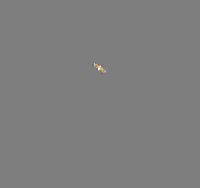## Recommended Posts

I want to make a particle moving randomly in the range of (-pi/9, pi/9) on a sphere, but the result I get is rotating around X axis, Is there anyone could help me with it? Thanks.

```#include "math.h"
pop
testpop()
{
vector   myP = normalize(P);
float   myrand = fit(random(Time),0,1,PI/-9,PI/9);
matrix  myident = ident();
matrix  myrot = rotate(myident,myrand,myP);
vector   myD = normalize(cross(v*myrot,myP));
P = normalize(P+myD*3)*30;
}
```

rotation.hipnc

##### Share on other sites

I want to make a particle moving randomly in the range of (-pi/9, pi/9) on a sphere, but the result I get is rotating around X axis, Is there anyone could help me with it? Thanks.

```#include "math.h"
pop
testpop()
{
vector   myP = normalize(P);
float   myrand = fit(random(Time),0,1,PI/-9,PI/9);
matrix  myident = ident();
matrix  myrot = rotate(myident,myrand,myP);
vector   myD = normalize(cross(v*myrot,myP));
P = normalize(P+myD*3)*30;
}
```

the problem seems be solved. Here is the new code:

```#include "math.h"
pop
testop()
{
vector   myP = normalize(P);
float   myrand = fit(random(Frame),0,1,PI/-9,PI/9);
matrix   myrot = ident();
myrot = rotate(myrot,myrand,myP);
vector   myV = normalize(cross(v*myrot,myP));
v *= myrot;
P = normalize(myP*20+myV) *5;
}
```

but still has a long way to go till the final result## Create an account or sign in to comment

You need to be a member in order to leave a comment

## Create an account

Sign up for a new account in our community. It's easy!

Register a new account